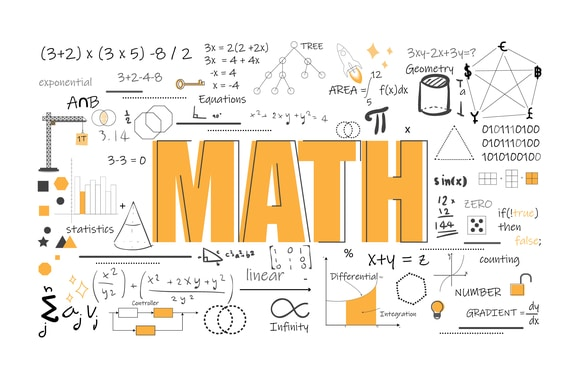# Factorization: Common Factors

Welcome to Class !!

In today’s Mathematics class, We will be talking about Factorization involving Common Factors. We hope you enjoy the class!#### FACTORIZATION: COMMON FACTORS

a(b + c) = ab + ac

The reverse process, ab + ac = a(b + c), is called taking out the common factor.

Consider the factorisation of the expression 5x + 15.

Clearly, 5x = 5 X x

15 = 3 X 5

∴ HCF = 5

Thus 5x + 15 = 5 X x + 3 X 5

= 5(x + 3)

Note that the common factor 5 has been taken out and placed in front of the brackets.  The expression inside the brackets is obtained by dividing each term by 5.

In general:

To factorise an algebraic expression, take out the highest common factor and place it in front of the brackets.  Then the expression inside the brackets is obtained by dividing each term by the highest common factor.

Example

Factorise the following

1. 15x – 20
2. 3px + 12qx
3. x+ x
4. 8x2y + 6xy2

Solution:

1) 15x – 20     {HCF = 5}

= 5(3x – 4)

2) 3px + 12qx     {HCF = 3x}

= 3x(p + 4q)

3) x+ x     {HCF = x}

= x(x + 1)

4) 8x2y + 6xy{HCF = 2xy}

= 2xy(4x + 3y)

Note: The process of taking out a common factor is of great importance in algebra.  With practice, you will be able to find the highest common factor (HCF) readily and hence factorise the given expression.

Example

Facrtorise the following:

1. -8x + 12
2. –x– x + ax
3. -6x+ 4x – 2px

Solution:

1) -8x

-8x + 12     {HCF = 4}

= 4(-2x + 3)

It is customary to have the first term in the brackets positive. So, we take out -4 as the common factor rather than 4.

So, -8x + 12 = -4(2x – 3)

2) –x– x + ax    {Take out –x as the common factor}

= -x(x + 1 – a)

3) -6x+ 4x – 2px      {Take out the common factor -2x}

= -2x(3x – 2 + p)

#### Simplifying calculations by factorization

Example

By factorizing, simplify 79 X 37 + 21 X 37.

37 is a common factor of 79 X 37 and 21 X 37.

79 X 37 + 21 X 37 = 37(79 + 21)

= 37 X 100

= 3,700

Factorise the expression πr+ 2πrh. Hence, find the value of πr+ 2πrh when π = 22/7, r = 14 and h = 43.

π r+2πrh = πr(r + 2h)

when π = 22/7, r = 14 and h = 43,

πr+ 2πrh = πr(r + 2h)

= 22/7 X 14(14 + 2 X 43)

= 22 X 2(14 + 86)

= 44 X 100

= 4400

#### Factorization by grouping

This section introduces the technique of factoring by grouping, as well as factoring the sum and difference of cubes.  Factoring by grouping builds on the ideas that were presented in the section on factoring the common factor.  While there are many different types of grouping, as you will learn in higher algebra courses, all of the grouping problems in this book involve four terms, and they work by grouping the first two terms and the second two terms together.   If you do it right, a common factor will always emerge!  Remember, the method of grouping is one of trial and error.  As always, there is no substitute for practice and experience. Remember, if you can learn this topic now, it will help you later.

Example

Factor  x+ 2x+ 8x + 16

Solution: There are no common factors to all four terms.  It is not a trinomial, and nothing discussed so far works to factor this.  So, try grouping the first two terms together, and the last two terms together, and factor out the common factor within each grouping as follows:

(x+ 2x2) + (8x + 16) =  x(x + 2)+ 8(x + 2)

Notice that there is a common factor of (x+2) that can be factored out:

=  (x + 2)( x+ 8)

Example

Factor  xy  4y + 3x  12

Solution:

Again, there are no common factors, and this is not a trinomial.  Group the first two and the last two terms together, and factor out the common factor from each grouping:

(xy  4y) + (3x  12) =  y(x − 4) + 3(x − 4)

Now, take out the common factor, which is (x − 4):

=  (x − 4)(y + 3)

Example

Factor  xy  4y  3x + 12

Solution:

Group the first two and the last two terms together, and factor out the common factor from each grouping:

(xy  4y) + (3x + 12) = y(x − 4) + 3(−x + 4)

This time there is no common factor.  Try again, this time factoring a 3 from the last grouping.  This works!

xy  4y   3x + 12 = y(x − 4) − 3(x − 4)

= (x − 4)(y  3)

Example

Factor  xy  4y + 3x + 12

Solution:

Group the first two and the last two terms:

xy  4y + 3x + 12 = y(x − 4) + 3(x + 4)

At this point, it is important to realize that no common factor resulted.  Do not try to factor out something that is not common to both groupings.  In fact, there is no way to group this problem to get a common factor.  This one cannot be factored by grouping.  In fact, it can not be factored by any method.  Remember, not all problems can be factored.  Remember that the entire process of grouping is one of trial and error, and, as you will see later, there are different types of grouping.

EVALUATION

1. Factorise the following: 4xy + 6zy,  kx – 6k – 9x + 24
2. Factorise 12mn + 8kn and solve for k if m=5 and n=2

We have come to the end of this class. We do hope you enjoyed the class?

Should you have any further question, feel free to ask in the comment section below and trust us to respond as soon as possible.

In our next class, we will be looking at the Factorization of Quadratic Equations. We are very much eager to meet you there.

Are you a Parent? Share your quick opinion and win free 2-month Premium Subscription

Don`t copy text!